Skip to content
Related Articles
Introduction to Trigonometric Ratios of a Triangle
• Last Updated : 31 Oct, 2020

Trigonometry is all about triangles or to be more precise about the relation between the angles and sides of a right-angled triangle. There are three sides of a triangles named as Hypotenuse, Adjacent, and Opposite. The ratio between these sides based on the angle between them are called Trigonometric Ratios.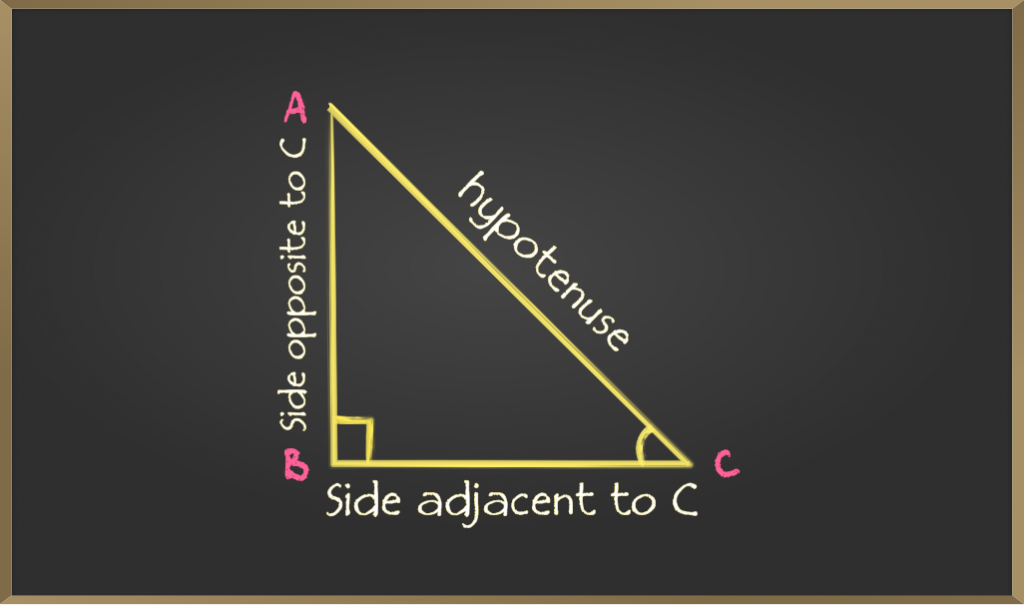As given in the figure in a right angle triangle

• The side opposite to the right angle is called the hypotenuse
• The side opposite to an angle is called the opposite side
• For angle C opposite side is AB
• For angle A opposite side is BC
• The side adjacent to an angle is called the adjacent side
• For angle C adjacent side is BC
• For angle A adjacent side is AB

### Trigonometric ratios

There are 6 basic trigonometric relations that form the basics of trigonometry. These 6 trigonometric relations are ratios of all the different possible combinations in a right-angled triangle.

These trigonometric ratios are called

• Sine
• Cosine
• Tangent
• Cosecant
• Secant
• Cotangent

The mathematical symbol θ is used to denote the angle.

A. Sine (sin)

Sine of an angle is defined by the ratio of lengths of sides which is opposite to the angle and the hypotenuse. It is represented as sinθ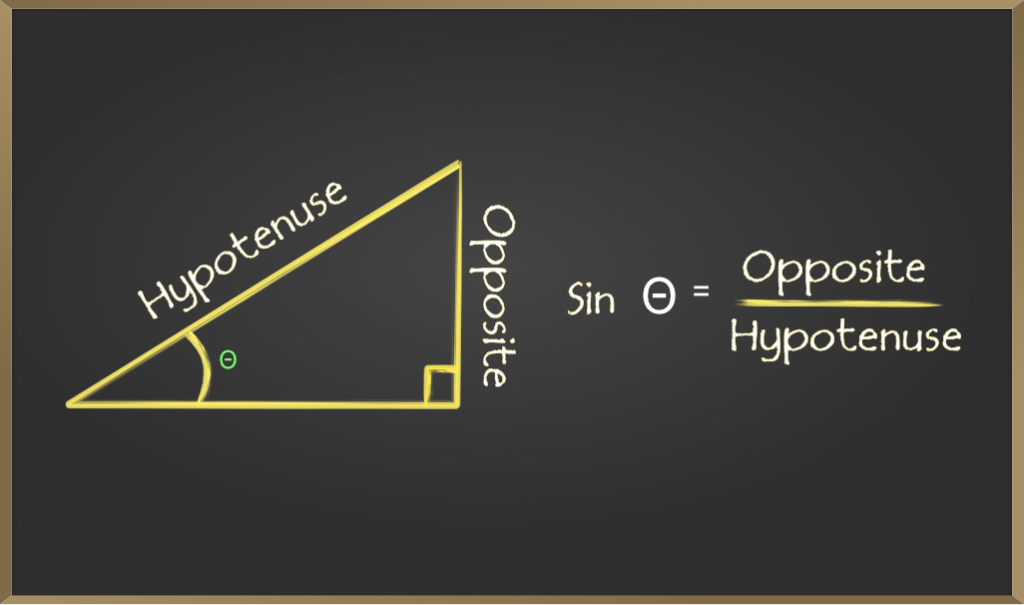B. Cosine (cos)

Cosine of an angle is defined by the ratio of lengths of sides which is adjacent to the angle and the hypotenuse. It is represented as cosθ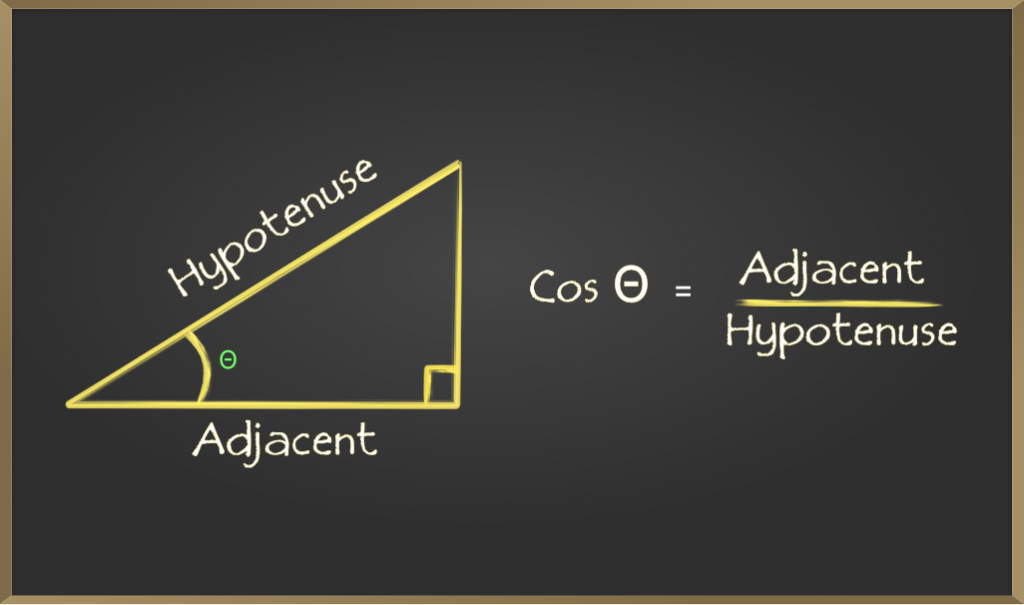C. Tangent(tan)

Tangent of an angle is defined by the ratio of length of sides which is opposite to the angle and the side which is adjacent to the angle. It is represented as tanθ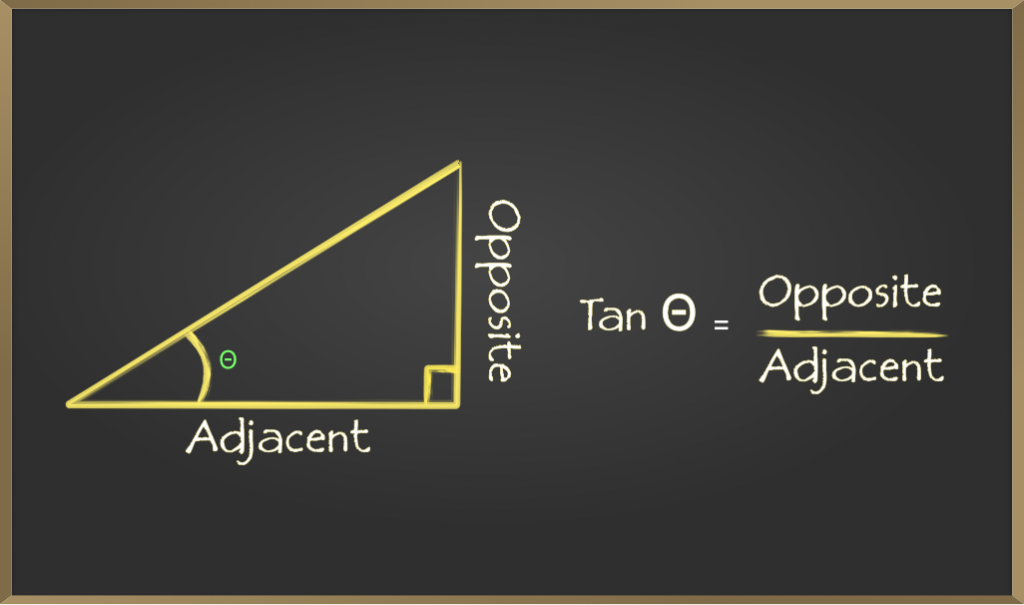D. Cosecant(csc)

Cosecent of an angle is defined by the ratio of length of the hypotenuse and the side opposite the angle. It is represented as cscθ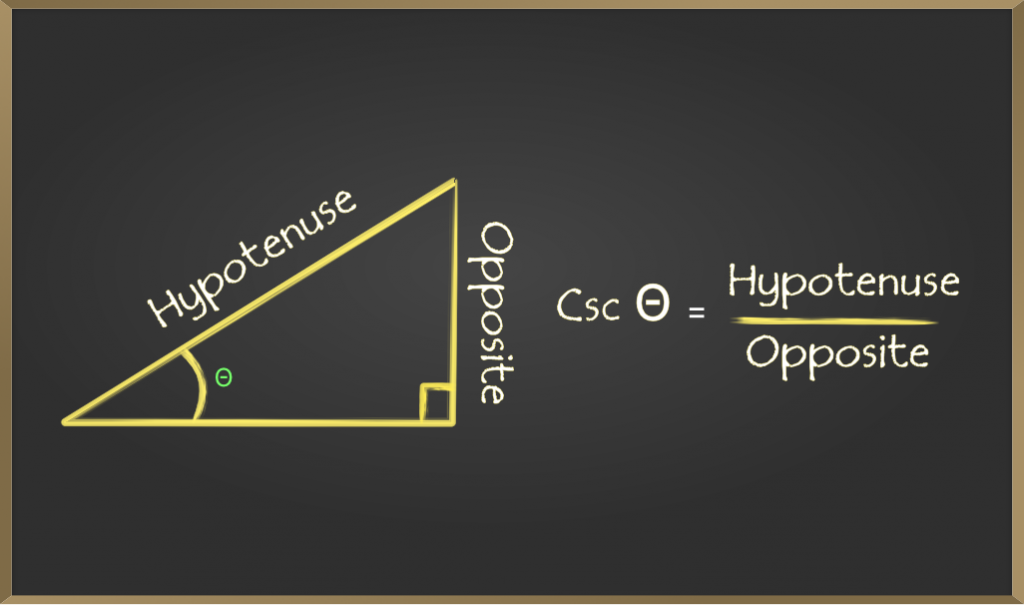E. Secant(sec)

Secant of an angle is defined by the ratio of length of the hypotenuse and the side and the side adjacent to the angle. It is represented as secθ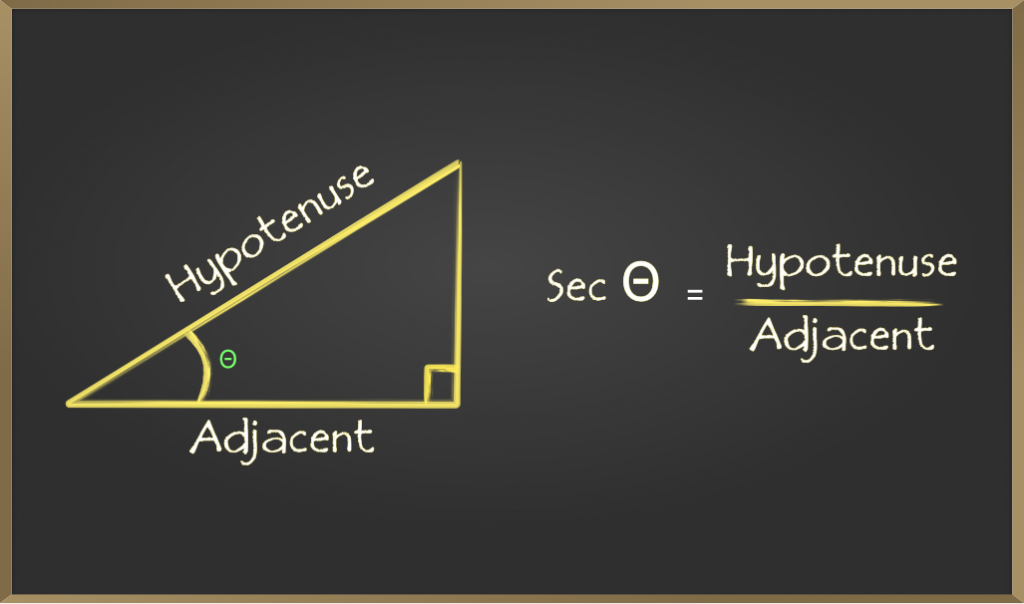F. Cotangent(cot)

Cotangent  of an angle is defined by the ratio of length of sides which is adjacent to the angle and the side which is opposite to the angle. It is represented as cotθ.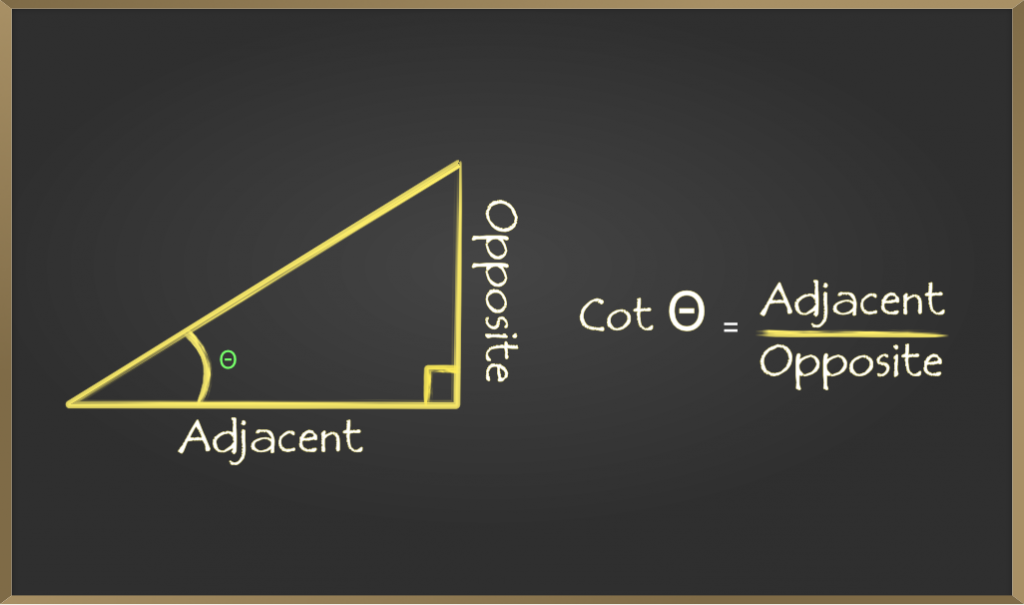### Solving for a side in right triangles with trigonometry

This is one of the most basic and useful use of trigonometry using the trigonometric ratios mentioned is to find the length of a side of a right-angled triangle But to do, so we must already know the length of the other two sides or an angle and length of one side.

Steps to follow if one side and one angle are known:

1. Choose a trigonometric ratio which contains the given side and the unknown side
2. Use algebra to find the unknown side

#### Example:Solution:Steps to follow if two sides are known:

1. Mark the known sides as adjacent, opposite or hypotenuse with respective to anyone of the acute angles in the triangle.
2. Decide on which trigonometric ratio can be found out from the above table.
3. Find the angle (X)
4. Use an trigonometric ratio with respect to X which is a ratio of a known side and an unknown side.
5. Use algebra to find the unknown side.

#### Example:Solution:My Personal Notes arrow_drop_up